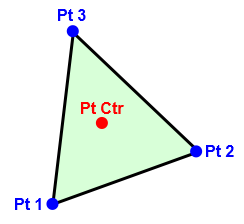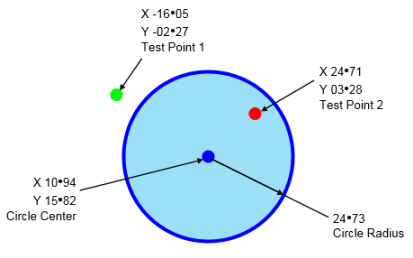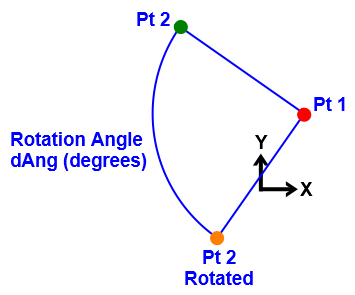It is currently Thu Jun 04, 2020 7:30 pm

 All times are UTC + 1 hourPage 4 of 4 [ 60 posts ] Go to page Previous  1, 2, 3, 4
 Print view Previous topic | Next topic
Author Message
 Post subject: Re: Game Math (Post all your snippits and guides here). ThanPosted: Thu Jun 09, 2016 2:57 pmEnthusiastJoined: Sun Jul 07, 2013 11:35 am
Posts: 515
IdeasVacuum wrote:
....nice one StarBootics, thanks for sharing. I'm surprised to see read/write file Procedures that do not need to Open/Create/Close the file.

You're welcome !

The way I'm writing my libraries I always create an instruction to Read/Write on binary file created somewhere else. If you check carefully it's true for Vector & Matrix libraries and not just for "Plane" calculation.
The beauty of open source code like these if something is missing, you can add it or if something is not useful you can remove it (comment the procedure or delete the code)

Best regards
StarBootics

_________________
The Stone Age did not end due to a shortage of stones !

TopPost subject: Re: Game Math (Post all your snippits and guides here). ThanPosted: Sat Jun 11, 2016 12:03 amEnthusiastJoined: Sun Jul 07, 2013 11:35 am
Posts: 515
Hello everyone,

This is an update (and some correction) of my Gaming Maths Library, featuring Vector2, Vector3, Vector4, Quaternion, Matrix22, Matrix33, Matrix34, Matrix44 and Plane. (Everything is coded into modules)
I have leave the Quaternion VS Matrix44 transformation demo. Matrix34 are still under development and it's use are for RigidBody simulation only.

https://www.dropbox.com/s/ei1cckqn22r9brh/Gaming_Maths_Library.zip?dl=0

Best regards
StarBootics

_________________
The Stone Age did not end due to a shortage of stones !

TopPost subject: Re: Game Math (Post all your snippits and guides here). ThanPosted: Mon Mar 04, 2019 5:07 pmEnthusiastJoined: Tue Apr 27, 2010 3:20 pm
Posts: 144
Location: Wales, United Kingdom
Wrapping a value between 0 - X

Useful if you need to, say, wrap an angle between 0 and 360;

Quote:
Procedure WrapValue(Value, Max = 360)
Value = Value % Max
If Value < 0
Value = Value + Max
EndIf
ProcedureReturn Value
EndProcedure

For X = -720 To 720
Debug WrapValue(X)
Next

_________________
Thanks!

TopPost subject: Re: Game Math (Post all your snippits and guides here). ThanPosted: Mon Apr 08, 2019 4:41 pmAlways HereJoined: Fri Oct 23, 2009 2:33 am
Posts: 6189
Location: Wales, UK
Wrap a float angle by Psychophanta:
Code:
Procedure.f WrapAngleDeg(angle.f)
;#-------------------------------
; <- wraps a value into [0,360) fringe
; Author : Psychophanta

!fild dword[@f] ; <- now i have 360 into st0
!fld dword[p.v_angle]
!fprem
!fldz
!fcomip st1
!fcmovnbe st0,st1
!fstp st1
ProcedureReturn
!@@:dd 360
EndProcedure

Does anyone know how to do it with doubles?

_________________
IdeasVacuum
If it sounds simple, you have not grasped the complexity.

TopPost subject: Re: Game Math (Post all your snippits and guides here). ThanPosted: Mon Apr 08, 2019 6:25 pmAddictJoined: Fri Apr 25, 2003 11:10 pm
Posts: 1223
very minor change
Code:
Procedure.d WrapAngleDeg(angle.d)
;#-------------------------------
; <- wraps a value into [0,360) fringe
; Author : Psychophanta

!fild dword[@f] ; <- now i have 360 into st0
!fld qword[p.v_angle] ;<- changed from dword to qword
!fprem
!fldz
!fcomip st1
!fcmovnbe st0,st1
!fstp st1
ProcedureReturn
!@@:dd 360
EndProcedure

TopPost subject: Re: Game Math (Post all your snippits and guides here). ThanPosted: Sat Apr 13, 2019 12:10 pmAlways HereJoined: Fri Oct 23, 2009 2:33 am
Posts: 6189
Location: Wales, UK
Ah yes, thanks Jack. I should have looked up the meaning of dword in assembly!

_________________
IdeasVacuum
If it sounds simple, you have not grasped the complexity.

TopPost subject: Re: Game Math (Post all your snippits and guides here). ThanPosted: Mon Dec 09, 2019 1:35 amAlways HereJoined: Fri Oct 23, 2009 2:33 am
Posts: 6189
Location: Wales, UK
Find the Centre of a Triangle (or Triangle Face)Code:
Procedure TriangleCtr(*PtCtr.pt3f, *Pt1.pt3f, *Pt2.pt3f, *Pt3.pt3f)
;#-----------------------------------------------------------------

*PtCtr\x = ( (*Pt1\x + *Pt2\x + *Pt3\x) / 3)
*PtCtr\y = ( (*Pt1\y + *Pt2\y + *Pt3\y) / 3)
*PtCtr\z = ( (*Pt1\z + *Pt2\z + *Pt3\z) / 3)
EndProcedure

_________________
IdeasVacuum
If it sounds simple, you have not grasped the complexity.

TopPost subject: Re: Game Math (Post all your snippits and guides here). ThanPosted: Thu Mar 12, 2020 10:36 amAddictJoined: Wed Jun 11, 2003 9:33 pm
Posts: 4623
Location: Spa, relaxing and thinking, and learning...
May be the best conversor for floating point standard notation number to the so called scientific notation number.Function + its testing:
Code:
Procedure\$ StrE(fp.d,dec.b=14)
;Devuelve cadena alfanumérica en formato de notación científica
Protected E.b,neg.b=0
If dec<0:dec=0:ElseIf dec>20:dec=20:EndIf;<- Sanity check
E=Round(Log10(Abs(fp)),#PB_Round_Nearest)
If E
fp=Round(fp*Pow(10.0,-E)*Pow(10.0,dec),#PB_Round_Nearest)/Pow(10.0,dec)
If fp>-1 And fp<1:fp*10.0:E-1:EndIf
If E:ProcedureReturn RTrim(RTrim(StrD(fp,dec),"0"),".")+"E"+Str(E):EndIf
EndIf
ProcedureReturn RTrim(RTrim(StrD(fp,dec),"0"),".")
EndProcedure

debug StrE(0.1)
debug StrE(0.01)
debug StrE(0.001)
debug StrE(0.0001)
debug StrE(0.00001)
debug StrE(1)
debug StrE(10)
debug StrE(100)
debug StrE(1000)
debug StrE(10000)
debug ""
debug "******"
debug ""
For i.u=1 to 1000
v.d=(Random(200000)-100000)/Random(1000000000)
debug strD(v,14)+"      ->      "+StrE(v,3)
v.d=(Random(20000000)-10000000)/Random(1000)
debug strD(v,14)+"      ->      "+StrE(v,3)
Next

_________________
http://www.zeitgeistmovie.com

TopPost subject: Re: Game Math (Post all your snippits and guides here). ThanPosted: Tue Apr 21, 2020 11:30 pmAlways HereJoined: Fri Oct 23, 2009 2:33 am
Posts: 6189
Location: Wales, UK
Is Point Inside CircleCode:
Structure pt2d
x.d
y.d
EndStructure

;#------------------------------------------------------------------------------
;Compare radius of circle with distance of its center to given point
Protected dX.d, dY.d, dZ.d, dC.d

dX = Abs(*CircleCtrPt\x - *TestPt\x)
dY = Abs(*CircleCtrPt\y - *TestPt\y)
dC = Sqr((dX * dX) + (dY * dY)) ;Point-To-Point distance

EndProcedure

;Example as per diagram
;----------------------
Define CircleCtrPt.pt2d
Define TestPt1.pt2d
Define TestPt2.pt2d

CircleCtrPt\x = 10.94
CircleCtrPt\y = 15.82

TestPt1\x = -16.05
TestPt1\y = -02.27

TestPt2\x = 24.71
TestPt2\y =  3.28

Edit: Improvement re Lord's suggestion

_________________
IdeasVacuum
If it sounds simple, you have not grasped the complexity.

Last edited by IdeasVacuum on Wed Apr 22, 2020 10:54 pm, edited 3 times in total.

TopPost subject: Re: Game Math (Post all your snippits and guides here). ThanPosted: Wed Apr 22, 2020 8:58 amEnthusiastJoined: Tue May 26, 2009 2:11 pm
Posts: 648
Hi!

For
Code:
...
ProcedureReturn(#True)
Else
ProcedureReturn(#False)
EndIf
...
this is also possible:
Code:
...
...

_________________TopPost subject: Re: Game Math (Post all your snippits and guides here). ThanPosted: Thu Apr 30, 2020 3:17 pmEnthusiastJoined: Sun Jul 07, 2013 11:35 am
Posts: 515
Post Deleted Considered Off-Topic

_________________
The Stone Age did not end due to a shortage of stones !

Last edited by StarBootics on Fri May 01, 2020 5:59 am, edited 3 times in total.

TopPost subject: Re: Game Math (Post all your snippits and guides here). ThanPosted: Thu Apr 30, 2020 6:18 pmEnthusiastJoined: Tue Sep 22, 2009 10:41 pm
Posts: 544
@Starbootics

If never you could use an other data space that dropbox : this last one is not reachable unhappily for me...

@IdeasVacuum

Code:
Procedure BelongToDisc(X.I, Y.I, DiscX.I, DiscY.I, DiscR.I) ; integer use constrains to a max of radius of 32000 on X86, 2400000000 on X64

DiscR * DiscR
X - DiscX
Y - DiscY
X * X
Y * Y

ProcedureReturn Bool(X + Y < DiscR)

EndProcedure

Code:
Procedure BelongToBall(X.I, Y.I, Z.I, DiscX.I, DiscY.I, DiscZ.I, DiscR.I) ; note integer use contrains to a max radius of 32000 on x86 and 2400000000 on X64

DiscR * DiscR
X - DiscX
Y - DiscY
Z - DiscZ
X * X
Y * Y
Z * Z

ProcedureReturn Bool(X + Y + Z < DiscR)

EndProcedure

Code:
Procedure BelongToHyperBall(W.I, X.I, Y.I, Z.I, DiscW.I, DiscX.I, DiscY.I, DiscZ.I, DiscR.I) ; note integer use contrains to a max radius of 32000 on x86 and 2400000000 on X64

DiscR * DiscR
W - DiscW
X - DiscX
Y - DiscY
Z - DiscZ
W * W
X * X
Y * Y
Z * Z

ProcedureReturn Bool(W + X + Y + Z < DiscR)

EndProcedure

TopPost subject: Re: Game Math (Post all your snippits and guides here). ThanPosted: Sun May 03, 2020 11:06 pmAlways HereJoined: Fri Oct 23, 2009 2:33 am
Posts: 6189
Location: Wales, UK
Rotate Point about Point by x degrees (diagram not to scale)Code:
Structure pt2d
x.d
y.d
EndStructure

Procedure RotatePt2d(*pt1.pt2d, *pt2.pt2d, dAng.d)
;#-----------------------------------------------
Protected dDx.d, dDy.d

dDx = (*pt2\x - *pt1\x)
dDy = (*pt2\y - *pt1\y)

EndProcedure

Define pt1.pt2d
Define pt2.pt2d

pt1\x = 20.57075  : pt1\y = 37.09869
pt2\x = -34.00713 : pt2\y = 64.56599

RotatePt2d(pt1, pt2, 90.00)

Debug pt2\x
Debug pt2\y

_________________
IdeasVacuum
If it sounds simple, you have not grasped the complexity.

TopPost subject: Re: Game Math (Post all your snippits and guides here). ThanPosted: Wed May 06, 2020 4:12 amUserJoined: Fri Jan 08, 2010 2:30 am
Posts: 18
Location: Oregon USA
I am not sure if anyone want a trajectory solver, but here is one that I wrote during research of 1900 vintage warships. Notes follow along with program listing. Trajectory3.pb I am not so familiar with posting to this forum, so if you need to give me pointers on how to improve, I will be thankful. Best Regards, St Paul.

Below, I offer a program that predicts range and impact conditions for naval cannon fired at known angles and initial velocities. DragFactor value of .25 to 3 may be used to adjust results to known ballistic solutions. Realistic values would be 1.0 to .75 and as low as about .5 for shells of the 1930s. This will ensure other solutions for the same projectile are correct. I run it on a Mac and made 10 or 11 trial runs to make sure it gets the same answers as my JavaScript version.

The solution method is a pretty standard numerical solution method as would be found in Engineering or Physics schools. However, the 1885 Bashforth Equation is used in lieu of modern day force equations. And yes, it produces correct results provided a correct Drag Factor DF is used.

Detailed Notes Follow:
1. Program designed to predict impact conditions for projectile fired with given initial conditions
2. Program is written in English units (inch, pounds, ft/sec, degrees etc.). My appologies
3. Recommended use is during game development. Code likely slow for active use.
I suggest curve fitting results applicable to your game for faster execution.
4. Initial balistic conditions are stated in lines 22 - 27
5. Output results are displayed in simple Debug statement at 106-115
6. Accumlation of flight path parameters for graphing commented out. 45-55 and 84-87
7. DF for 1890 Brit shells = .75. A table on my web site provides typical values for other nations and time periods. Not sure if I am allowed to post that here. But I can answer questions.
8. DF is your method for "tunning" the analysis to match a known result. Then all other predictions should be pretty good.
9. Calculation method uses Bashforth 1885 historical equation. This turns out to be rougly equivalent to modern methods, except frictional factor kbashforth is a bit different and a v^3 friction law is used to compensate for changes in k. The equation was developed for naval cannon from 3 inch to 12 inch. It should work fine for 1 inch up to 20 or 24 inches. Velocities should be less than 3000 feet/sec for this method. I have tested this equation for many conditions and found it to predict reasonably accurate results. Also, I added a correction for air density at altitude which was not needed in 1885 as ranges were short and high angle fire was not used. As corrected, you should get good answers, even with high angle mortars.
10. This same program should work with arrows, boulders from catapults, spears etc. I have not found the friction coefficients for these, so I am not sure what drag factor DF to use. We can figure something out if someone has a need. The easiest solution is to identify one well known test point where weight, initial velocity, firing angle and terminal range are known. Then trial and error to find the matching DF should allow future solutions at different firing angles and initial velocities.
11. I can answer questions if any on this forum.

Code:
; program computes terminal conditions of projectile fired at given initial conditions
; Use 1885 Bashforth equation for to determine air friction
Global Dim bash.f(3)
Procedure Bashforth(v,d,w,theta,y,DF)
; establish friction coefficient as function of gross speed. Should not be broken into x & y components
If v<1061.999
k.f=18120.39461*Pow(v,-0.8082372052) ;Bashforth Friction Coefficient k below 1062 ft/sec
Else
k.f=2.9e-8*Pow(v,3)-1.5924e-4*Pow(v,2)+0.213533*v+52.75734 ;Bashforth Friction Coefficient k above 1062 ft/sec
EndIf

densityFactor.f=1.0098095*Pow(2.718281828,-0.00003206*y); Atmospheric density as funct of y has been curve fit
k=DF*densityFactor*k ; overall friction factor k is product of drag factor, air density and k
Ax.f=k*Pow(d,2)*Pow(v,3)*Cos(theta/57.3)/(1e9*w); x component of acceleration
Ay.f=k*Pow(d,2)*Pow(v,3)*Sin(theta/57.3)/(1e9*w); y component of acceleration
bash(0)=k ; an array for return value
bash(1)=Ax
bash(2)=Ay
EndProcedure

; initial conditions
w.f=0.0 ; default is 850 pounds
d.f=14.0  ; default is 12 inches
yInit.f=20 ; default firing is at deck height of 20 feet
vInit.f=2500.0 ; use muzzel velocity in ft/sec
DF.f=0.6     ; assumed Drag Factor = 1.0
thetaInit.f=25.0 ; default barrel elevation is 12 degrees

If vInit>3000.0 ; equations break down ovr 3000 ft/sec
vInit=3000.0
EndIf
If d>24.0 ; equation may break down over 24 inches bore size
d=24.0
EndIf
If w=0.0
w=0.5*Pow(d,3) ; default weight of shells good for 1890 through 1960
EndIf
If DF<0.25 Or DF>3.0 ; unrealistic drag factors outside range .25 to 3.0 for cannon shells
DF=1.0
EndIf
If thetaInit<0.5
thetaInit=0.5
EndIf

; array declarations use values below if you want to trap the flight profile
;Dim timeRecord(500)
;Dim xRecord(500)
;Dim yRecord(500)
;Dim SpeedRecord(500)
;Dim thetaRecord(500)
; xRecord(0)=0.0
; yRecord(0)=0.0
; timeRecord(0)=0.0
; thetaRecord(0)=theta1
; SpeedRecord(0)=vInit

; establish initial x & y velocities
X1.f=0
Y1.f=yInit
Vx1.f=vInit*Cos(thetaInit/57.3)
Vy1.f=vInit*Sin(thetaInit/57.3)
V1.f=vInit
theta1.f=thetaInit ; this is in degrees
Tdelta.f=0.2
time.f=0.0

; loop to step through time at .2 sec intervals
For i=0 To 400
bashforth(V1,d,w,theta1,y1,DF)
k.f=bash(0)
Ax.f=bash(1)
Ay.f=bash(2)
Vx2.f=Vx1-Ax*Tdelta
Vy2.f=Vy1-Ay*Tdelta-32*Tdelta
X2=X1+Vx2*Tdelta
Y2=Y1+Vy2*Tdelta

V2=Sqr(Pow(Vx2,2)+Pow(Vy2,2))

theta2.f=57.3*ATan((Y2-Y1)/(X2-X1)); in degrees
temp1=Y2-Y1; did I ever declar this as float?
temp2=X2-X1; ditto

;timeRecord(i)=time use these values if you want to trap flight profile
;xRecord(i)=X2
;yRecord(i)=Y2
;thetaRecord(i)=theta2

; set old values to new
time=time+Tdelta
X1=X2
Y1=Y2
Vx1=Vx2
Vy1=Vy2
V1=V2
theta1=theta2
If Y1<0
Break
EndIf
Next i

angFinal=-1*theta2
DI.f=30/Tan(angFinal/57.3)

Debug "Range in ft ="
Debug X2
Debug "Velocity at impact ft/sec"
Debug V2
Debug "Angle of impact deg"
Debug angFinal
Debug "Flight Time sec"
Debug time
Debug "Danger Interval ft"
Debug 30/Tan(angFinal/57.3)
End

// Edit: Code tags added. (Kiffi)

_________________
[img]StPaul.jpg/img]

TopPost subject: Re: Game Math (Post all your snippits and guides here). ThanPosted: Wed May 06, 2020 5:40 amUserJoined: Fri Jan 08, 2010 2:30 am
Posts: 18
Location: Oregon USA
Trajectory solver code for above comment. Just figured out about the code block entry. So here it is:

Code:
; program computes terminal conditions of projectile fired at given initial conditions
; Use 1885 Bashforth equation for to determine air friction
Global Dim bash.f(3)
Procedure Bashforth(v,d,w,theta,y,DF)
; establish friction coefficient as function of gross speed. Should not be broken into x & y components
If v<1061.999
k.f=18120.39461*Pow(v,-0.8082372052) ;Bashforth Friction Coefficient k below 1062 ft/sec
Else
k.f=2.9e-8*Pow(v,3)-1.5924e-4*Pow(v,2)+0.213533*v+52.75734 ;Bashforth Friction Coefficient k above 1062 ft/sec
EndIf

densityFactor.f=1.0098095*Pow(2.718281828,-0.00003206*y); Atmospheric density as funct of y has been curve fit
k=DF*densityFactor*k ; overall friction factor k is product of drag factor, air density and k
Ax.f=k*Pow(d,2)*Pow(v,3)*Cos(theta/57.3)/(1e9*w); x component of acceleration
Ay.f=k*Pow(d,2)*Pow(v,3)*Sin(theta/57.3)/(1e9*w); y component of acceleration
bash(0)=k ; an array for return value
bash(1)=Ax
bash(2)=Ay
EndProcedure

; initial conditions
w.f=0.0 ; default is 850 pounds
d.f=14.0  ; default is 12 inches
yInit.f=20 ; default firing is at deck height of 20 feet
vInit.f=2500.0 ; use muzzel velocity in ft/sec
DF.f=0.6     ; assumed Drag Factor = 1.0
thetaInit.f=25.0 ; default barrel elevation is 12 degrees

If vInit>3000.0 ; equations break down ovr 3000 ft/sec
vInit=3000.0
EndIf
If d>24.0 ; equation may break down over 24 inches bore size
d=24.0
EndIf
If w=0.0
w=0.5*Pow(d,3) ; default weight of shells good for 1890 through 1960
EndIf
If DF<0.25 Or DF>3.0 ; unrealistic drag factors outside range .25 to 3.0 for cannon shells
DF=1.0
EndIf
If thetaInit<0.5
thetaInit=0.5
EndIf

; array declarations use values below if you want to trap the flight profile
;Dim timeRecord(500)
;Dim xRecord(500)
;Dim yRecord(500)
;Dim SpeedRecord(500)
;Dim thetaRecord(500)
; xRecord(0)=0.0
; yRecord(0)=0.0
; timeRecord(0)=0.0
; thetaRecord(0)=theta1
; SpeedRecord(0)=vInit

; establish initial x & y velocities
X1.f=0
Y1.f=yInit
Vx1.f=vInit*Cos(thetaInit/57.3)
Vy1.f=vInit*Sin(thetaInit/57.3)
V1.f=vInit
theta1.f=thetaInit ; this is in degrees
Tdelta.f=0.2
time.f=0.0

; loop to step through time at .2 sec intervals
For i=0 To 400
bashforth(V1,d,w,theta1,y1,DF)
k.f=bash(0)
Ax.f=bash(1)
Ay.f=bash(2)
Vx2.f=Vx1-Ax*Tdelta
Vy2.f=Vy1-Ay*Tdelta-32*Tdelta
X2=X1+Vx2*Tdelta
Y2=Y1+Vy2*Tdelta

V2=Sqr(Pow(Vx2,2)+Pow(Vy2,2))

theta2.f=57.3*ATan((Y2-Y1)/(X2-X1)); in degrees
temp1=Y2-Y1; did I ever declar this as float?
temp2=X2-X1; ditto

;timeRecord(i)=time use these values if you want to trap flight profile
;xRecord(i)=X2
;yRecord(i)=Y2
;thetaRecord(i)=theta2

; set old values to new
time=time+Tdelta
X1=X2
Y1=Y2
Vx1=Vx2
Vy1=Vy2
V1=V2
theta1=theta2
If Y1<0
Break
EndIf
Next i

angFinal=-1*theta2
DI.f=30/Tan(angFinal/57.3)

Debug "Range in ft ="
Debug X2
Debug "Velocity at impact ft/sec"
Debug V2
Debug "Angle of impact deg"
Debug angFinal
Debug "Flight Time sec"
Debug time
Debug "Danger Interval ft"
Debug 30/Tan(angFinal/57.3)
End

// Edit: Code tags added. (Kiffi)

_________________
[img]StPaul.jpg/img]

TopDisplay posts from previous: All posts1 day7 days2 weeks1 month3 months6 months1 year Sort by AuthorPost timeSubject AscendingDescendingPage 4 of 4 [ 60 posts ] Go to page Previous  1, 2, 3, 4

 All times are UTC + 1 hour

#### Who is online

Users browsing this forum: No registered users and 15 guests

 You cannot post new topics in this forumYou cannot reply to topics in this forumYou cannot edit your posts in this forumYou cannot delete your posts in this forum

Search for:
 Jump to:  Select a forum ------------------ PureBasic    Coding Questions    Game Programming    3D Programming    Assembly Programming    The PureBasic Editor    The PureBasic Form Designer    General Discussion    Feature Requests and Wishlists    Tricks 'n' Tips Bug Reports    Bugs - Windows    Bugs - Linux    Bugs - Mac OSX    Bugs - IDE    Bugs - Documentation OS Specific    AmigaOS    Linux    Windows    Mac OSX Miscellaneous    Announcement    Off Topic Showcase    Applications - Feedback and Discussion    PureFORM & JaPBe    TailBite